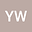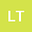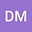Multiple Solutions for a Kirchhoff-type Fractional Coupled Problem with p-Laplacian
•••• yi wany,
• Lixin Tian,
• Dong Min-Jie
yi wany
School of Mathematical Sciences

Corresponding Author:wy182270@163.com

Author ProfileLixin Tian
School of Mathematical Sciences
Author ProfileDong Min-Jie
School of physical and mathematical sciences
Author Profile## Abstract

In this paper, we look at a class of two-parameter coupled Kirchhoff-type fractional differential equations. Two differentiated methods are used to prove the existence of two solutions to the equation. The fundamental difference between the two methods is that the first provides asymptotic conditions for the non-linear terms on the right-hand side of the equation, while the second provides algebraic conditions; both methods combine substantial A-R conditions.